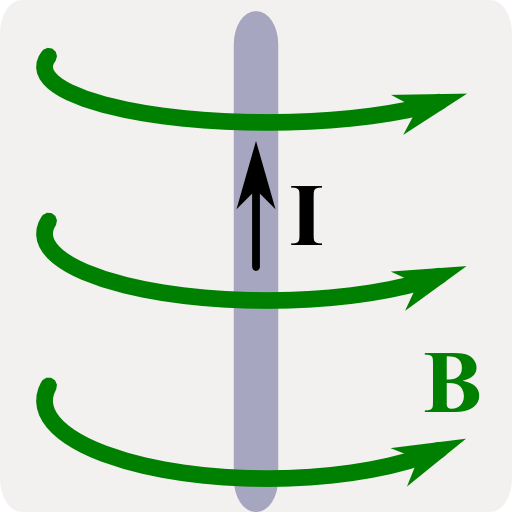## The Magnetic Field due to a Thin Infinite WireWe will directly use the Biot-Savart law to calculate the magnetic field of a thin wire via integration. The problem shall provide us with some intuition of Biot-Savart which we will also derive.

## Problem StatementIn The Magnetic Field of an Infinite Wire we discuss the field of a not necessarily thin wire with a constant current $$j_{0}\mathbf{e}_{z}$$ and discuss generalizations $$j_{0}\rightarrow j\left(\rho\right)$$. We made our calculations directly from the differential form of Ampère's law. If we assume a very thin wire, i.e. a current of the form$\mathbf{j}\left(\mathbf{r}\right) = I\delta\left(\rho\right)\mathbf{e}_{z}$in cylindrical coordinates, we may also calculate the magnetic field by direct integration via Biot-Savart's law,$\mathbf{B}\left(\mathbf{r}\right) = \frac{\mu_{0}I}{4\pi}\int\frac{d\mathbf{s}\times\left(\mathbf{r}-\mathbf{r}\left(s\right)\right)}{\left|\mathbf{r}-\mathbf{r}\left(s\right)\right|^{3}}\ ,$where the integral is over the curve of the thin wire.

• Calculate the magnetic field $$\mathbf{B}\left(\mathbf{r}\right)$$ for the thin wire, infinitely extended in $$z$$ using Biot-Savart law.
• Extra: Derive the Biot-Savart law from the connection of the vector potential to the current.